Home
Hostname: page-component-78bd46657c-jcldq Total loading time: 0.183 Render date: 2021-05-08T11:10:30.569Z Has data issue: true Feature Flags: { "shouldUseShareProductTool": true, "shouldUseHypothesis": true, "isUnsiloEnabled": true, "metricsAbstractViews": false, "figures": false, "newCiteModal": false, "newCitedByModal": true }Compositio Mathematica

# Self-dual integral normal bases and Galois module structure

Published online by Cambridge University Press:  10 May 2013

Corresponding

## Abstract

Let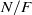$N/ F$ be an odd-degree Galois extension of number fields with Galois group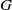$G$ and rings of integers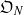${\mathfrak{O}}_{N}$ and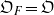${\mathfrak{O}}_{F} = \mathfrak{O}$. Let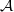$\mathcal{A}$ be the unique fractional${\mathfrak{O}}_{N}$-ideal with square equal to the inverse different of$N/ F$. B. Erez showed that$\mathcal{A}$ is a locally free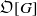$\mathfrak{O}[G]$-module if and only if$N/ F$ is a so-called weakly ramified extension. Although a number of results have been proved regarding the freeness of$\mathcal{A}$ as a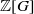$\mathbb{Z} [G]$-module, the question remains open. In this paper we prove that$\mathcal{A}$ is free as a$\mathbb{Z} [G]$-module provided that$N/ F$ is weakly ramified and under the hypothesis that for every prime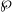$\wp$ of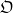$\mathfrak{O}$ which ramifies wildly in$N/ F$, the decomposition group is abelian, the ramification group is cyclic and$\wp$ is unramified in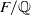$F/ \mathbb{Q}$. We make crucial use of a construction due to the first author which uses Dwork’s exponential power series to describe self-dual integral normal bases in Lubin–Tate extensions of local fields. This yields a new and striking relationship between the local norm-resolvent and the Galois Gauss sum involved. Our results generalise work of the second author concerning the case of base field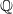$\mathbb{Q}$.

## MSC classification

Type
Research Article
Information
Compositio Mathematica , July 2013 , pp. 1175 - 1202

## References

Cassou-Noguès, P. and Taylor, M. J., Opérations d’Adams et groupe des classes d’algèbre de groupe, J. Algebra 95 (1985), 125152.CrossRefGoogle Scholar
Dwork, B., On the zeta functions of a hypersurface. II, Ann. of Math. (2) 80 (1964), 227299.CrossRefGoogle Scholar
Erez, B., The Galois structure of the square root of the inverse different, Math. Z. 208 (1991), 239255.CrossRefGoogle Scholar
Fesenko, I. B. and Vostokov, S. V., Local fields and their extensions, second edition (American Mathematical Society, Providence, RI, 2002).Google Scholar
Fröhlich, A., Galois module structure of algebraic integers (Springer, Berlin, 1983).CrossRefGoogle Scholar
Iwasawa, K., Local class field theory (Oxford University Press, Oxford, 1986).Google Scholar
Lang, S., Cyclotomic fields II (Springer, New York, 1980).CrossRefGoogle Scholar
Martinet, J., Character theory and Artin$L$-functions, in Algebraic number fields: L-functions and Galois properties, Proceedings of a Symposium at the University of Durham, 1975 (Academic Press, London, 1977), 187.Google Scholar
Pickett, E. J., Explicit construction of self-dual integral normal bases for the square-root of the inverse different, J. Number Theory 129 (2009), 17731785.CrossRefGoogle Scholar
Pickett, E. J., Construction of self-dual integral normal bases in abelian extensions of finite and local fields, Int. J. Number Theory 6 (2010), 15651588.CrossRefGoogle Scholar
Serre, J. P., Local class field theory, in Algebraic number theory, eds Cassels, J. W. S. and Fröhlich, A. (Academic Press, London, 1967).Google Scholar
Serre, J. P., Corps locaux (Hermann, Paris, 1968).Google Scholar
Tate, J. T., Local constants, in Algebraic number fields: L-functions and Galois properties, Proceedings of a Symposium at the University of Durham, 1975 (Academic Press, London, 1977), 89131, Prepared in collaboration with C. J. Bushnell and M. J. Taylor.Google Scholar
Taylor, M. J., On Fröhlich’s conjecture for rings of integers of tame extensions, Invent. Math. 63 (1981), 4179.CrossRefGoogle Scholar
Vinatier, S., Structure galoisienne dans les extensions faiblement ramifiées de$\mathbb{Q}$, J. Number Theory 91 (2001), 126152.CrossRefGoogle Scholar
Vinatier, S., Galois module structure in weakly ramified 3-extensions, Acta Arith. 119 (2005), 171186.CrossRefGoogle ScholarYou have Access

# Send article to Kindle

Note you can select to send to either the @free.kindle.com or @kindle.com variations. ‘@free.kindle.com’ emails are free but can only be sent to your device when it is connected to wi-fi. ‘@kindle.com’ emails can be delivered even when you are not connected to wi-fi, but note that service fees apply.

Find out more about the Kindle Personal Document Service.

Self-dual integral normal bases and Galois module structure
Available formats
×

# Send article to Dropbox

To send this article to your Dropbox account, please select one or more formats and confirm that you agree to abide by our usage policies. If this is the first time you use this feature, you will be asked to authorise Cambridge Core to connect with your <service> account. Find out more about sending content to Dropbox.

Self-dual integral normal bases and Galois module structure
Available formats
×

# Send article to Google Drive

To send this article to your Google Drive account, please select one or more formats and confirm that you agree to abide by our usage policies. If this is the first time you use this feature, you will be asked to authorise Cambridge Core to connect with your <service> account. Find out more about sending content to Google Drive.

Self-dual integral normal bases and Galois module structure
Available formats
×
×